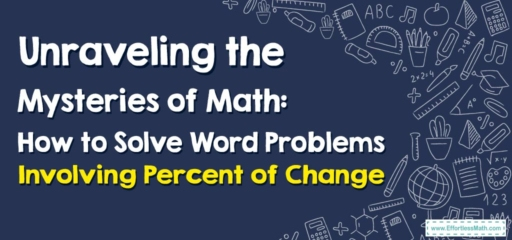# Unraveling the Mysteries of Math: How to Solve Word Problems Involving Percent of Change

Hello, young mathematicians!

Today, we're going to embrace the beauty of change, specifically the percent of change in mathematics. Math is not about numbers alone; it's about understanding the patterns, changes, and relationships among these numbers. So, let's explore this fascinating topic together!## 1. The Essence: Understanding Percent of Change

Before we dive in, let’s clarify what we mean by percent of change:

• Percent of Change: This refers to the ratio of the change in a quantity to the original quantity, expressed as a percentage. It helps us to understand how much a quantity has increased or decreased relative to its original value.

## 2. The Journey: Solving Word Problems Involving Percent of Change

Are you ready to venture into this wonderful world of percent changes? Let’s go!

### Mentor’s Guide: Solving Word Problems Involving Percent of Change

#### Step 1: Identify the Initial and Final Values

First, pinpoint the initial (original) and final (new) values given in the problem.

#### Step 2: Compute the Amount of Change

Next, calculate the change by subtracting the initial value from the final value.

#### Step 3: Divide by the Initial Value

After that, divide the amount of change by the initial value to determine the proportion of the change.

#### Step 4: Convert to Percentage

Lastly, convert the decimal to a percentage by multiplying it by $$100$$$$\%$$.

For instance, suppose your weekly allowance increased from $$10$$ to $$15$$. What is the percent of change?

1. Identify the Initial and Final Values: The initial allowance is $$10$$, and the final allowance is $$15$$.
2. Compute the Amount of Change: The change is $$15\ – 10 = 5$$.
3. Divide by the Initial Value: The proportion of change is $$\frac{5}{10} = 0.5$$.
4. Convert to Percentage: The percent of change is $$0.5\times100$$$$\%$$$$= 50$$$$\%$$.

So, there you have it, dear mathematicians! You’ve successfully journeyed through the realm of percent of change. Always remember, math is not just about getting the correct answer, but about understanding the why and how. Keep exploring and let your mathematical curiosity lead you onwards!

### What people say about "Unraveling the Mysteries of Math: How to Solve Word Problems Involving Percent of Change - Effortless Math: We Help Students Learn to LOVE Mathematics"?

No one replied yet.

X
30% OFF

Limited time only!

Save Over 30%

SAVE $5 It was$16.99 now it is \$11.99